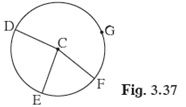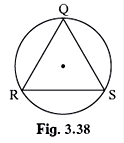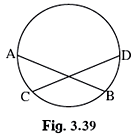SSC BOARD PAPERS IMPORTANT TOPICS COVERED FOR BOARD EXAM 2024

### Practice Set 3.3 Circle Class 10th Mathematics Part 2 MHB Solution

Practice Set 3.3

In figure 3.37, points G, D, E, F are concyclic points of a circle with centre C.∠ ECF…

In fig 3.38 ∆ QRS is an equilateral triangle. Prove that,(1) arc RS ≅ arc QS ≅ arc…

In fig 3.39 chord AB ≅ chord CD, Prove that, arc AC ≅ arc BD

###### Practice Set 3.3

Question 1.

In figure 3.37, points G, D, E, F are concyclic points of a circle with centre C.

∠ ECF = 70°, m(arc DGF) = 200° find m(arc DE) and m(arc DEF).Given ∠ECF = 70° and m(arc DGF) = 200°

We know that measure of major arc = 360° - measure of minor arc

m(arc DGF) = 360° - m(arc DF)

⇒ m(arc DF) = 360° - 200° = 160°

⇒∠ DCF = 160°

∵ The measure of a minor arc is the measure of its central angle.

∴m(arc DEF) = 160°

So, ∠DCE = ∠ DCF -∠ECF = 160° - 70°

⇒ ∠DCE = 90°

The measure of a minor arc is the measure of its central angle.

m(arc DE) = 90°

Question 2.

In fig 3.38 ∆ QRS is an equilateral triangle. Prove that,

(1) arc RS ≅ arc QS ≅ arc QR

(2) m(arc QRS) = 240°.(1) Two arcs are congruent if their measures and radii are equal.

∵∆ QRS is an equilateral triangle

∴ RS = QS = QR

⇒arc RS ≅ arc QS ≅ arc QR

(2) Let O be the centre of the circle.

m(arc QS) = ∠ QOS

∠ QOS + ∠ QOR + ∠ SOR = 360°

⇒ 3∠ QOS = 360° {∵ ∆QRS is an equilateral triangle}

⇒∠ QOS = 120°

m(arc QS) = 120°

m(arc QRS ) = 360° - 120° {∵Measure of a major arc = 360°- measure of its corresponding minor arc}

⇒m(arc QRS ) = 240°

Question 3.

In fig 3.39 chord AB ≅ chord CD, Prove that, arc AC ≅ arc BD∵ chord AB ≅ chord CD

∴ m(arc AB) = m(arc CD){Corresponding arcs of congruent chords of a circle (or congruent circles) are congruent}

Subtract m(arc CB) from above,

m(arc AB)– m(arc CB) = m(arc CD) – m(arc CB)

⇒m(arc AC) = m(arc BD)

⇒arc AC ≅ arc BD

Hence, proved.